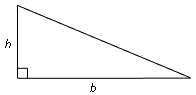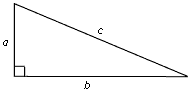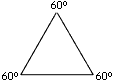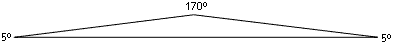### Excerpt from MathFacts™ I: Everything You Always Wanted to Know About Triangles

The Bermuda Triangle has nothing on the rest of the triangles in the world. Just as ships have allegedly disappeared in its watery jaws, so have many students allegedly disappeared in the three angles and three sides of garden-variety triangles, and they have been heard to moan in agony at the very words "isosceles" and "equilateral."

Relax, mates. You are about to learn all you need to know about triangles.

First off, a triangle is a two-dimensional (flat) figure with three angles and thus three sides (the tri- prefix is your tip-off; it means "three" just as it does in the word "tricycle"). Since it's 2-dimensional, we can compute area:The area of a triangle is 1/2bh, where b = the length and h = the height.

This formula works for any kind of triangle, as you may see by the examples below:These two triangles are identical in area; both measure 10 square inches.

Now, suppose you want to compute the lengths of the sides of a right triangle (and I guarantee you there will be a point during the SAT when this desire will befall you). Let's take an example which has a shortcut that can save you precious minutes during the exam. The Pythagorean Theorem says that the sum of the squares of the lengths of the two shortest sides of a right triangle is equal to the square of the length of the hypotenuse, which is the side opposite the right angle (also known as the 90-degree angle):a 2 + b 2 = c 2: if you add together the squares of the two shorter side lengths, you get the square of the hypotenuse.

Now comes the fancy part that saves you time on the SAT! Suppose the test reveals to you that a = 3 and b = 4. Do you have to use the formula above to compute the answer? No! You get to pass GO and collect \$200 because you've taken this course, where you've learned the following shortcut:

If a = 3 and b = 4, then c = 5.
If a = 6 and b = 8, then c = 10.
If a = 9 and b =12, then c = 15.

(Do you see a pattern?) This works for every right triangle with every multiple of 3, 4 & 5. So, if a = 30,639, and b = 40, 852, then c = 51,065; you don't need to calculate squares and square roots, and thus you save time for the tough questions.

What about equilateral triangles? If you know your Word Building and Discovery stuff, you see that "equi-" means "equal" and "lateral" goes back to the Latin for "side." So an equilateral triangle is one where all three sides are the same length. This also means that all three angles are the same size. Now, since there are always (in every plane-geometry triangle everywhere on the face of planet Earth) 180 degrees total for all three angles, there must be 60 degrees in each angle in an equilateral triangle (180 divided by 3 = 60).An equilateral triangle

The three angles of a triangle always add up to 180 degrees. This is true even if one of those angles has 170 degrees in it, in which case the remaining two have ten degrees to share, and we have a very skinny elongated triangle:A very strange triangle (probably not drawn to scale) with one angle having 170 degrees and the other two angles having but 5 degrees apiece.

This site will look MUCH better in a browser that supports web standards, but its content is accessible to any browser or Internet device.